Скачать презентацию Chapter 15 Optimization Modeling Applications Integer Programming

933a3dc7b783bfc9f1a11a11596e19f1.ppt

• Количество слайдов: 67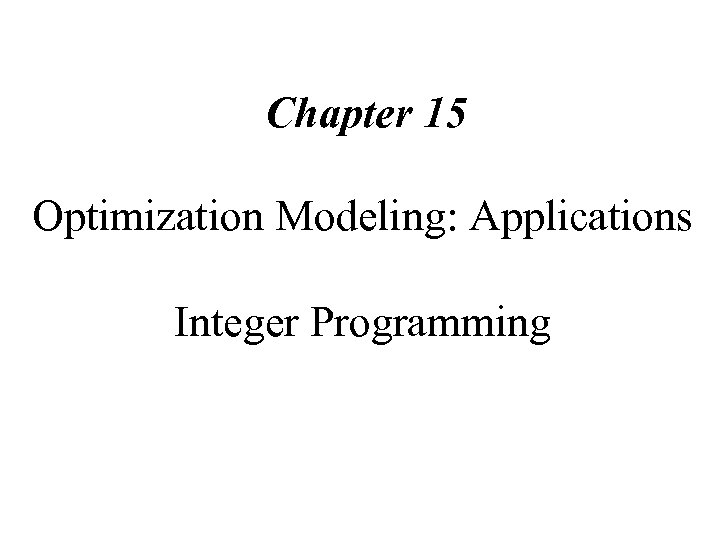Chapter 15 Optimization Modeling: Applications Integer Programming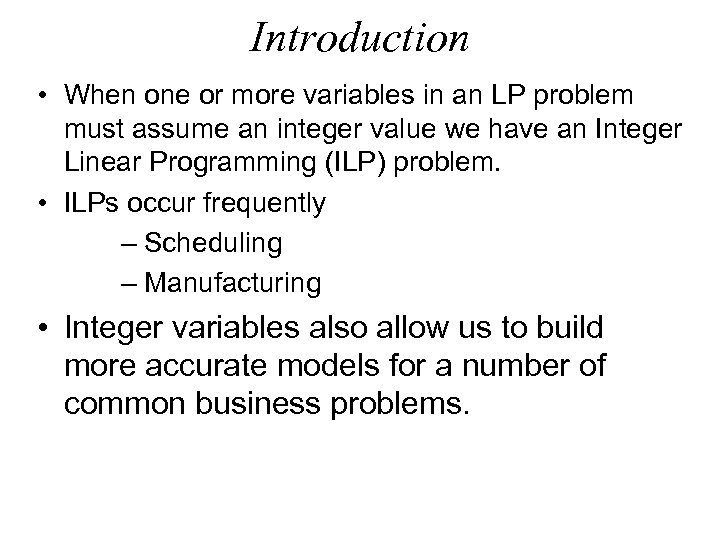Introduction • When one or more variables in an LP problem must assume an integer value we have an Integer Linear Programming (ILP) problem. • ILPs occur frequently – Scheduling – Manufacturing • Integer variables also allow us to build more accurate models for a number of common business problems.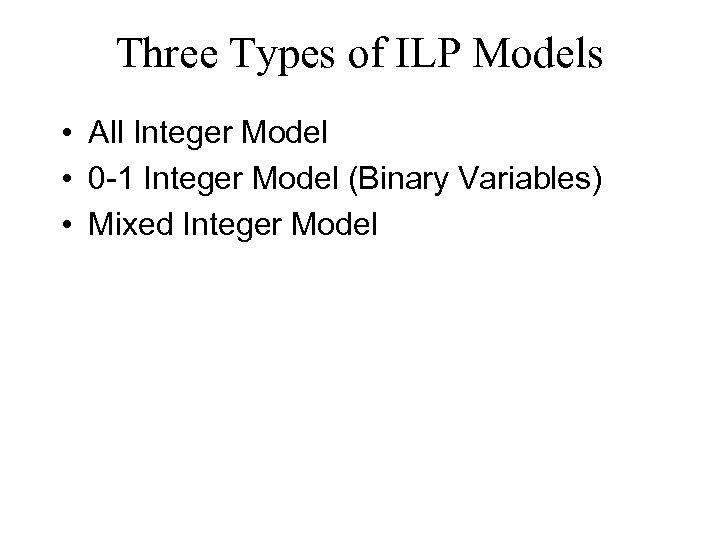Three Types of ILP Models • All Integer Model • 0 -1 Integer Model (Binary Variables) • Mixed Integer Model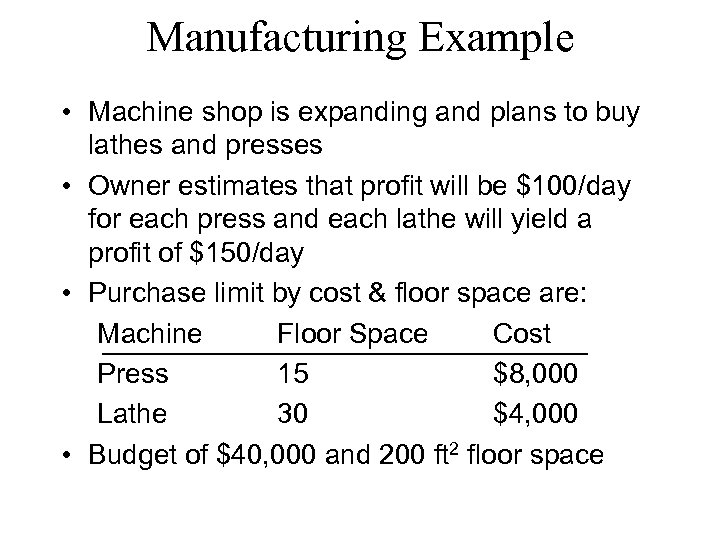Manufacturing Example • Machine shop is expanding and plans to buy lathes and presses • Owner estimates that profit will be \$100/day for each press and each lathe will yield a profit of \$150/day • Purchase limit by cost & floor space are: Machine Floor Space Cost Press 15 \$8, 000 Lathe 30 \$4, 000 • Budget of \$40, 000 and 200 ft 2 floor space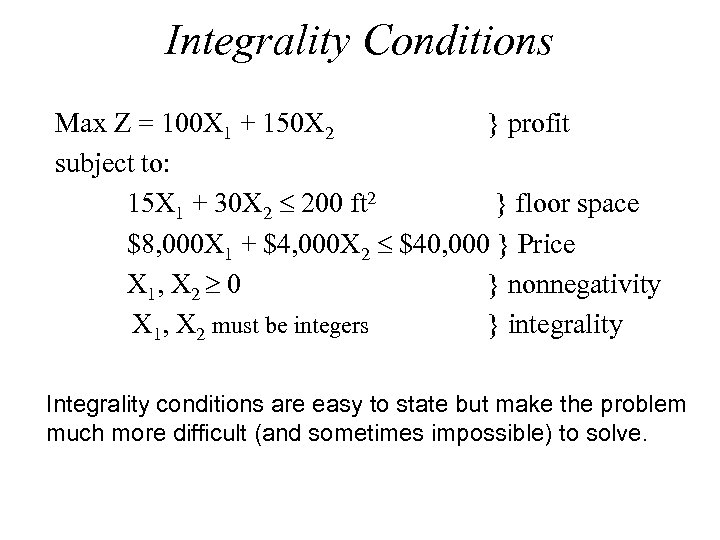Integrality Conditions Max Z = 100 X 1 + 150 X 2 } profit subject to: 15 X 1 + 30 X 2 200 ft 2 } floor space \$8, 000 X 1 + \$4, 000 X 2 \$40, 000 } Price X 1, X 2 0 } nonnegativity X 1, X 2 must be integers } integrality Integrality conditions are easy to state but make the problem much more difficult (and sometimes impossible) to solve.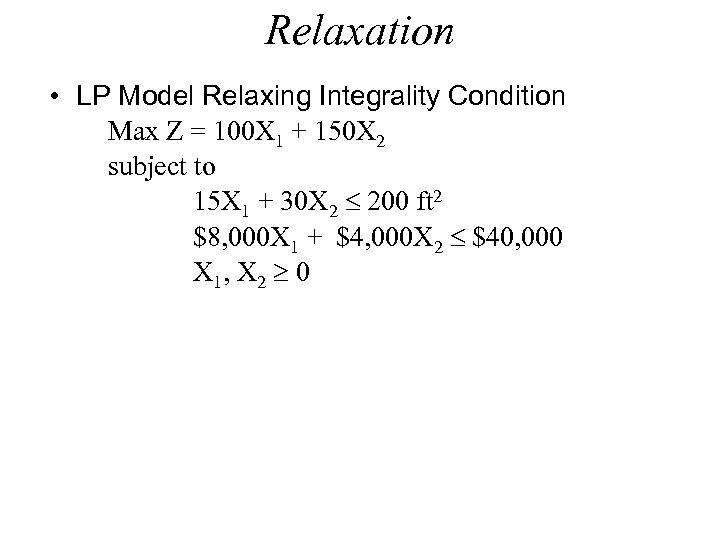Relaxation • LP Model Relaxing Integrality Condition Max Z = 100 X 1 + 150 X 2 subject to 15 X 1 + 30 X 2 200 ft 2 \$8, 000 X 1 + \$4, 000 X 2 \$40, 000 X 1, X 2 0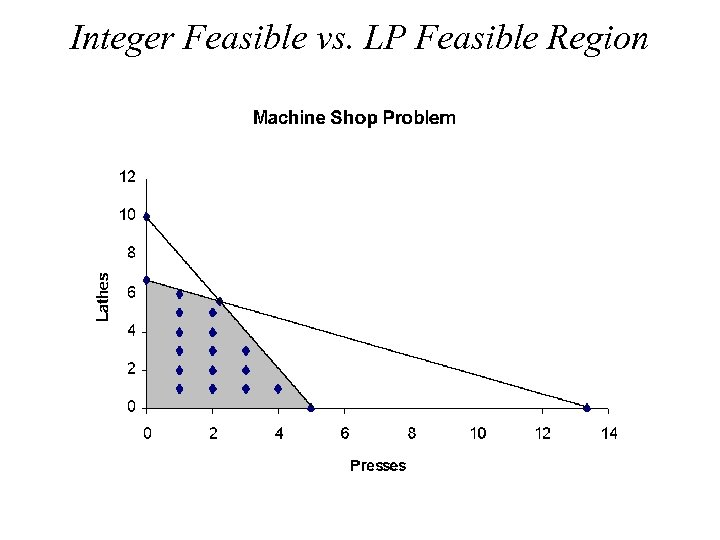Integer Feasible vs. LP Feasible Region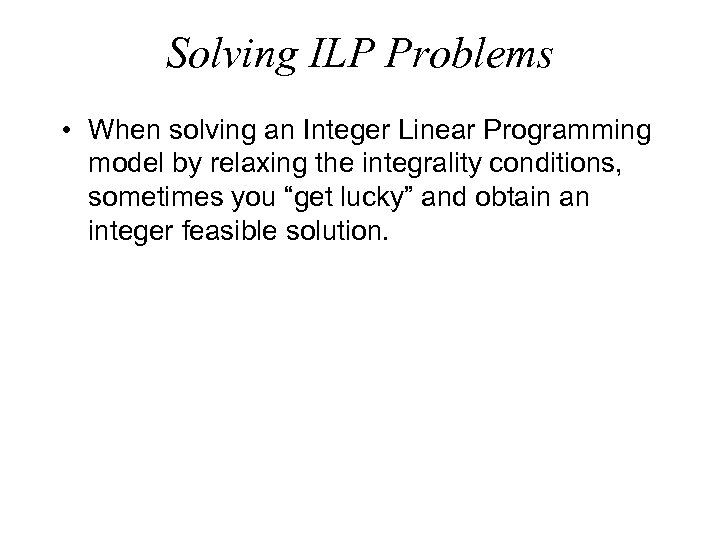Solving ILP Problems • When solving an Integer Linear Programming model by relaxing the integrality conditions, sometimes you “get lucky” and obtain an integer feasible solution.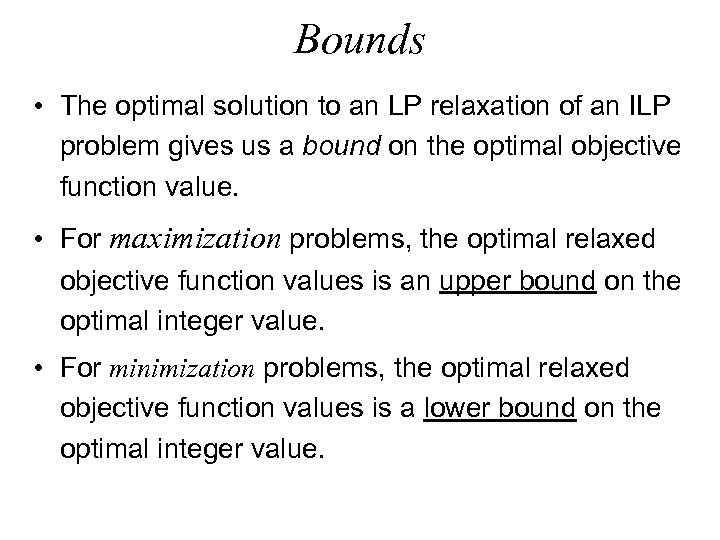Bounds • The optimal solution to an LP relaxation of an ILP problem gives us a bound on the optimal objective function value. • For maximization problems, the optimal relaxed objective function values is an upper bound on the optimal integer value. • For minimization problems, the optimal relaxed objective function values is a lower bound on the optimal integer value.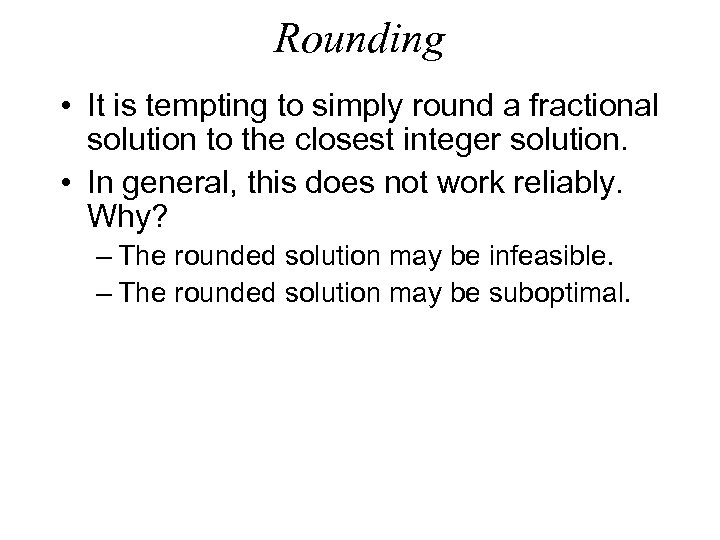Rounding • It is tempting to simply round a fractional solution to the closest integer solution. • In general, this does not work reliably. Why? – The rounded solution may be infeasible. – The rounded solution may be suboptimal.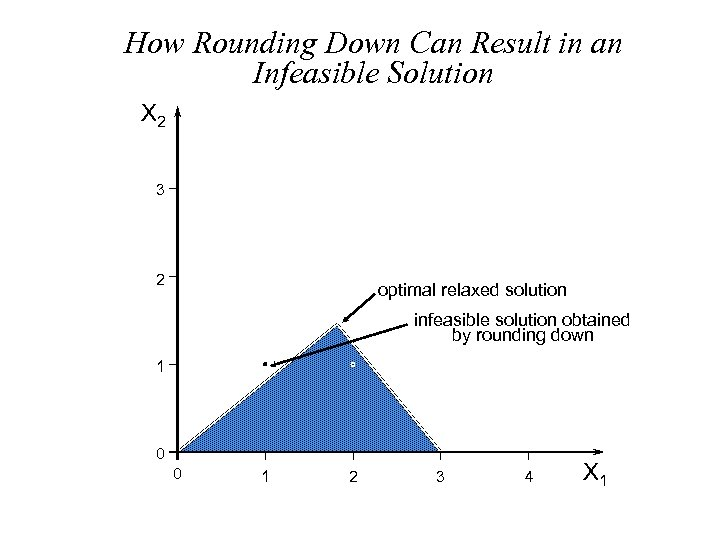How Rounding Down Can Result in an Infeasible Solution X 2 3 2 optimal relaxed solution infeasible solution obtained by rounding down 1 0 0 1 2 3 4 X 1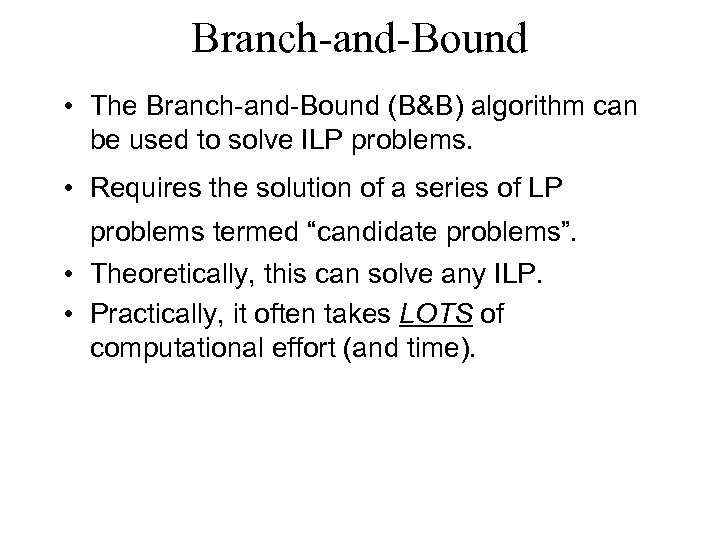Branch-and-Bound • The Branch-and-Bound (B&B) algorithm can be used to solve ILP problems. • Requires the solution of a series of LP problems termed “candidate problems”. • Theoretically, this can solve any ILP. • Practically, it often takes LOTS of computational effort (and time).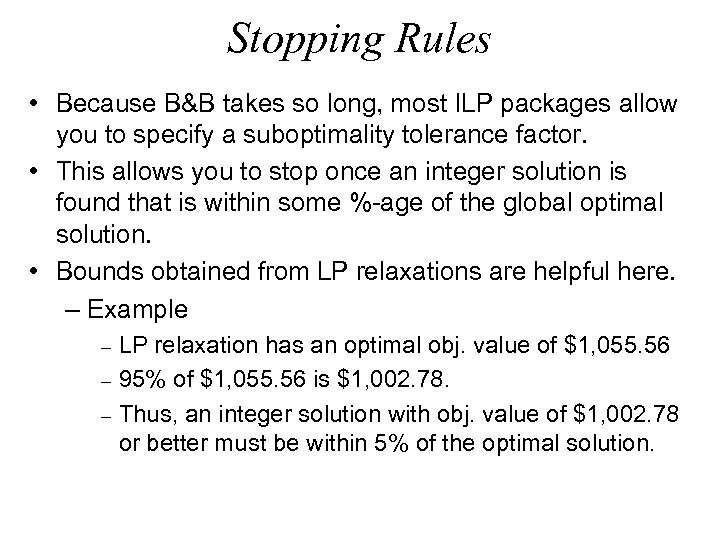Stopping Rules • Because B&B takes so long, most ILP packages allow you to specify a suboptimality tolerance factor. • This allows you to stop once an integer solution is found that is within some %-age of the global optimal solution. • Bounds obtained from LP relaxations are helpful here. – Example - LP relaxation has an optimal obj. value of \$1, 055. 56 95% of \$1, 055. 56 is \$1, 002. 78. Thus, an integer solution with obj. value of \$1, 002. 78 or better must be within 5% of the optimal solution.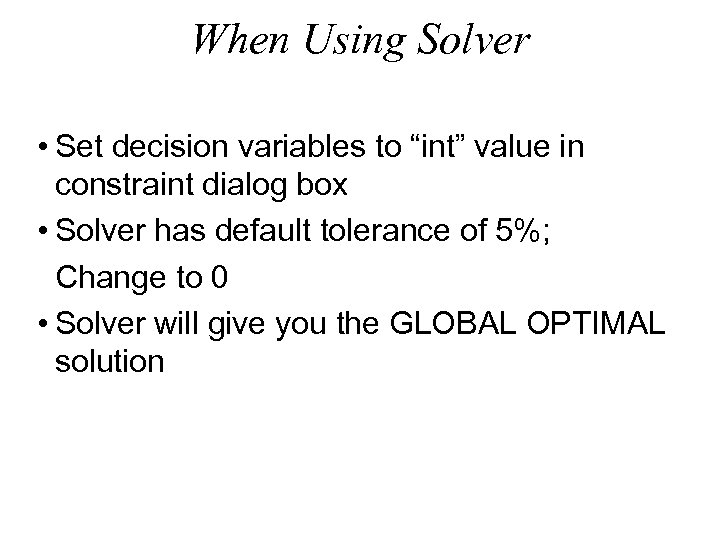When Using Solver • Set decision variables to “int” value in constraint dialog box • Solver has default tolerance of 5%; Change to 0 • Solver will give you the GLOBAL OPTIMAL solution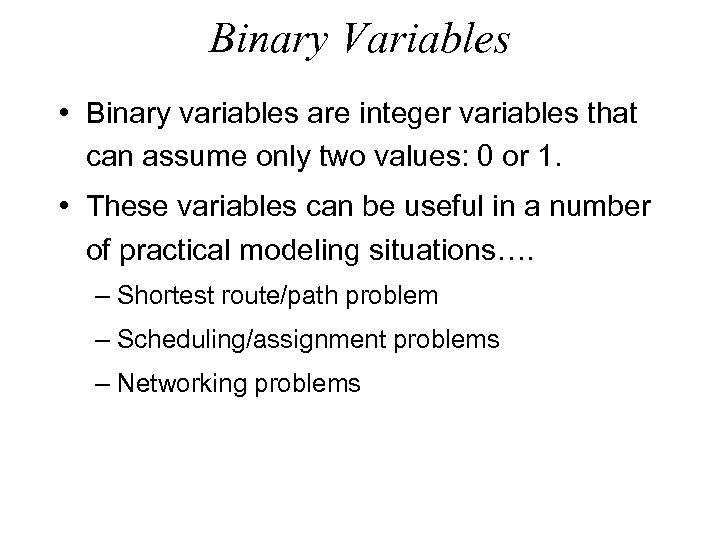Binary Variables • Binary variables are integer variables that can assume only two values: 0 or 1. • These variables can be useful in a number of practical modeling situations…. – Shortest route/path problem – Scheduling/assignment problems – Networking problems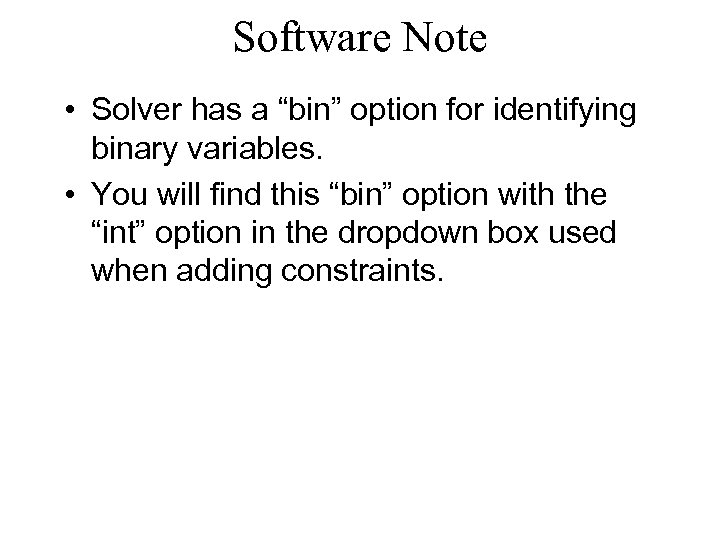Software Note • Solver has a “bin” option for identifying binary variables. • You will find this “bin” option with the “int” option in the dropdown box used when adding constraints.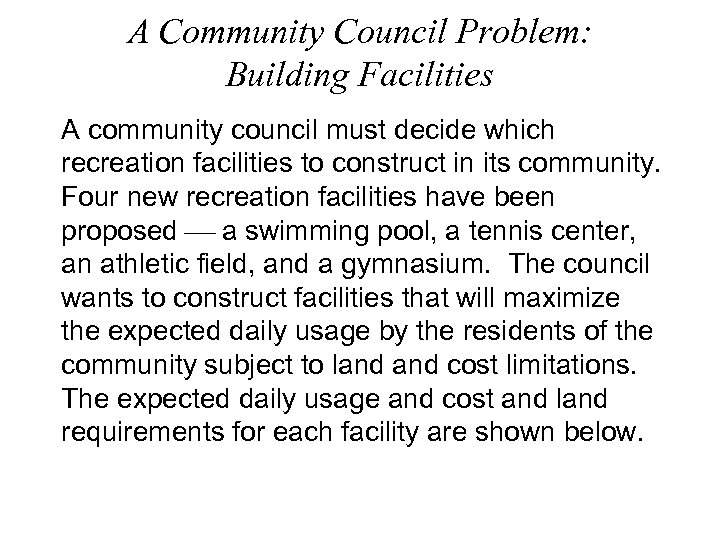A Community Council Problem: Building Facilities A community council must decide which recreation facilities to construct in its community. Four new recreation facilities have been proposed a swimming pool, a tennis center, an athletic field, and a gymnasium. The council wants to construct facilities that will maximize the expected daily usage by the residents of the community subject to land cost limitations. The expected daily usage and cost and land requirements for each facility are shown below.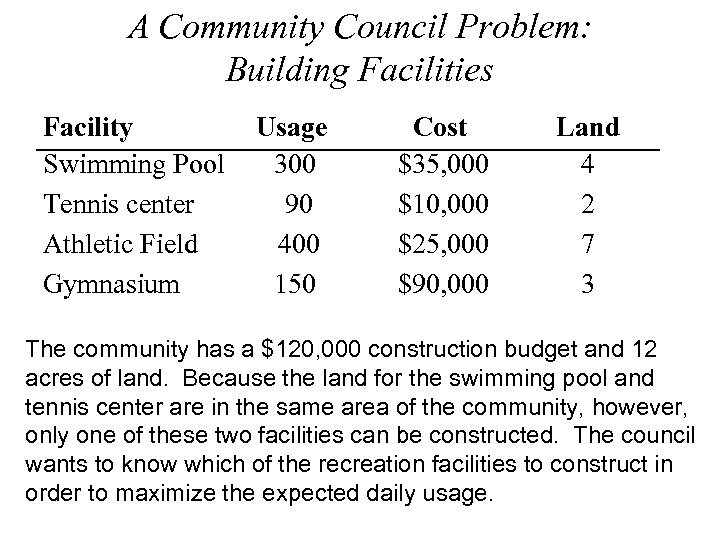A Community Council Problem: Building Facilities Facility Swimming Pool Tennis center Athletic Field Gymnasium Usage 300 90 400 150 Cost \$35, 000 \$10, 000 \$25, 000 \$90, 000 Land 4 2 7 3 The community has a \$120, 000 construction budget and 12 acres of land. Because the land for the swimming pool and tennis center are in the same area of the community, however, only one of these two facilities can be constructed. The council wants to know which of the recreation facilities to construct in order to maximize the expected daily usage.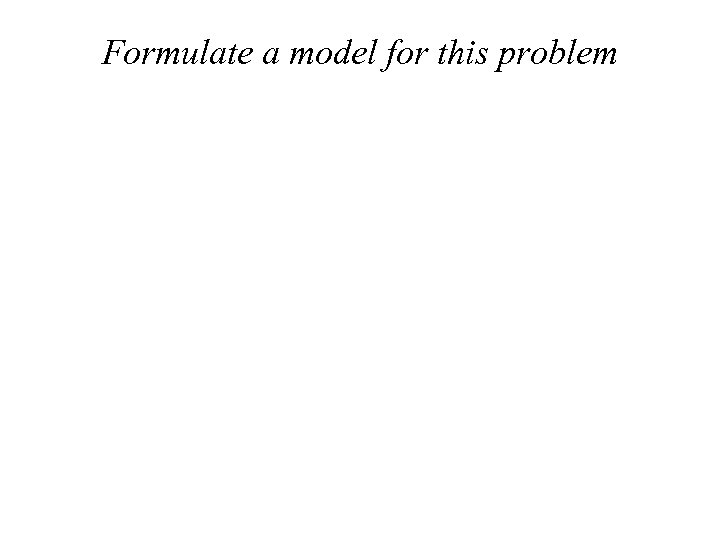Formulate a model for this problem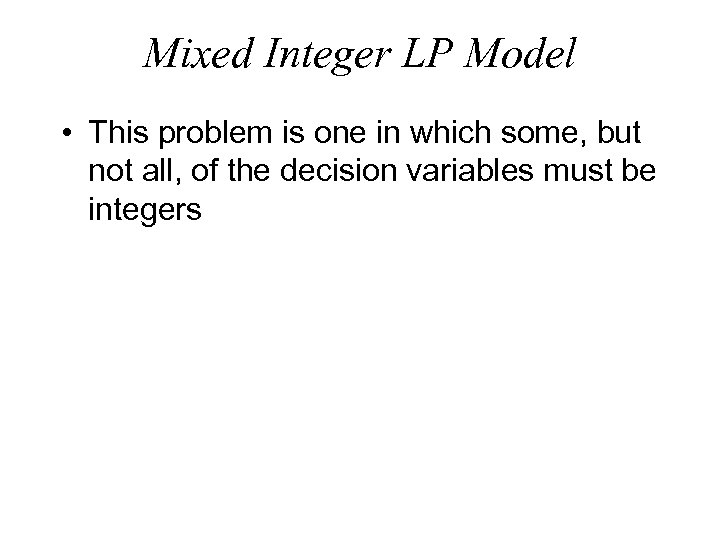Mixed Integer LP Model • This problem is one in which some, but not all, of the decision variables must be integers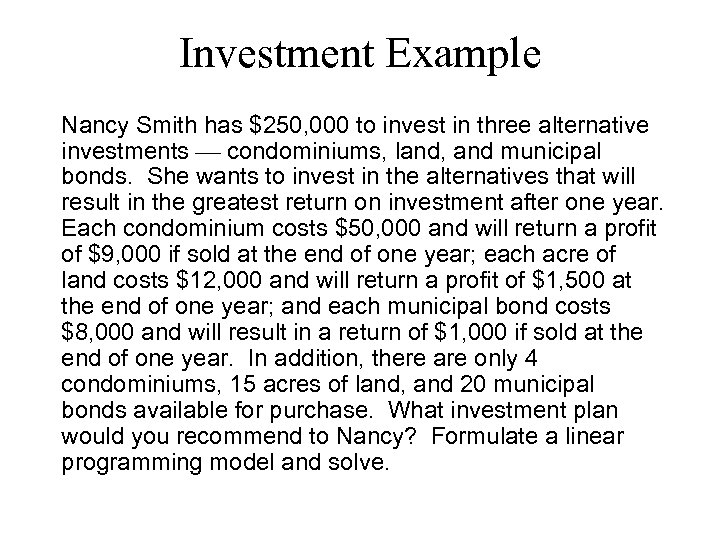Investment Example Nancy Smith has \$250, 000 to invest in three alternative investments condominiums, land, and municipal bonds. She wants to invest in the alternatives that will result in the greatest return on investment after one year. Each condominium costs \$50, 000 and will return a profit of \$9, 000 if sold at the end of one year; each acre of land costs \$12, 000 and will return a profit of \$1, 500 at the end of one year; and each municipal bond costs \$8, 000 and will result in a return of \$1, 000 if sold at the end of one year. In addition, there are only 4 condominiums, 15 acres of land, and 20 municipal bonds available for purchase. What investment plan would you recommend to Nancy? Formulate a linear programming model and solve.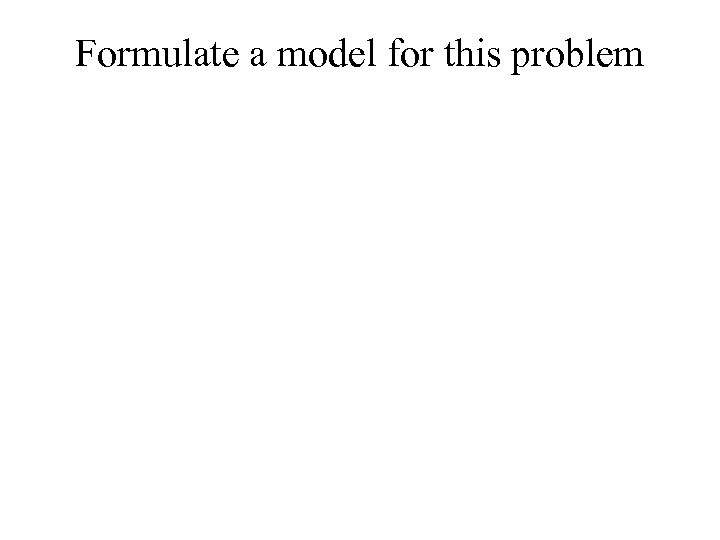Formulate a model for this problem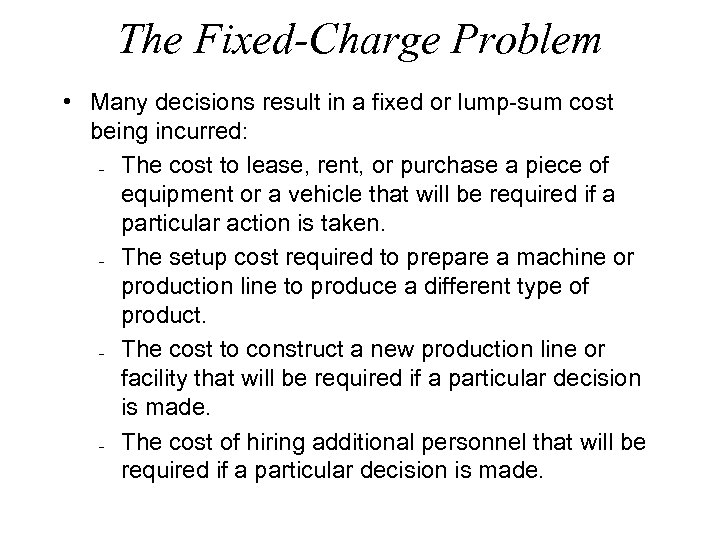The Fixed-Charge Problem • Many decisions result in a fixed or lump-sum cost being incurred: The cost to lease, rent, or purchase a piece of equipment or a vehicle that will be required if a particular action is taken. The setup cost required to prepare a machine or production line to produce a different type of product. The cost to construct a new production line or facility that will be required if a particular decision is made. The cost of hiring additional personnel that will be required if a particular decision is made.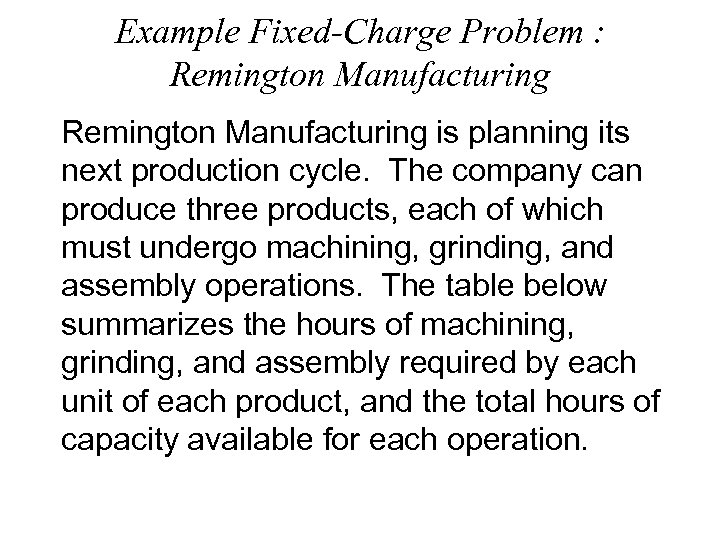Example Fixed-Charge Problem : Remington Manufacturing is planning its next production cycle. The company can produce three products, each of which must undergo machining, grinding, and assembly operations. The table below summarizes the hours of machining, grinding, and assembly required by each unit of each product, and the total hours of capacity available for each operation.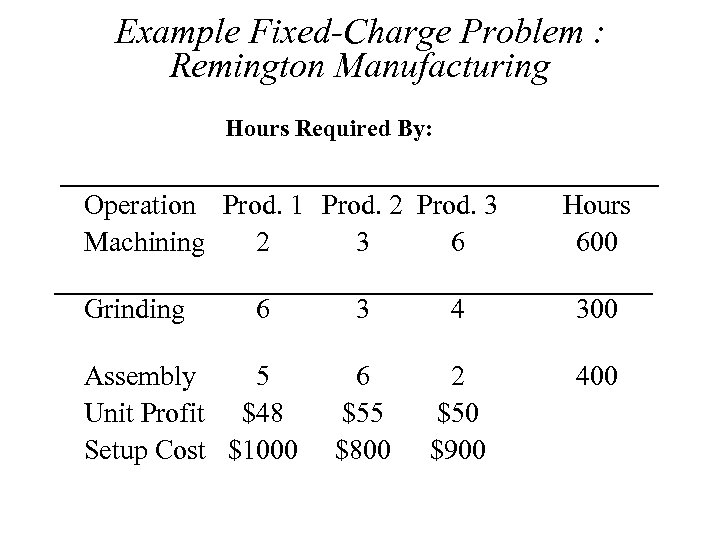Example Fixed-Charge Problem : Remington Manufacturing Hours Required By: Operation Prod. 1 Prod. 2 Prod. 3 Machining 2 3 6 Grinding 6 Assembly 5 Unit Profit \$48 Setup Cost \$1000 Hours 600 3 4 300 6 \$55 \$800 2 \$50 \$900 400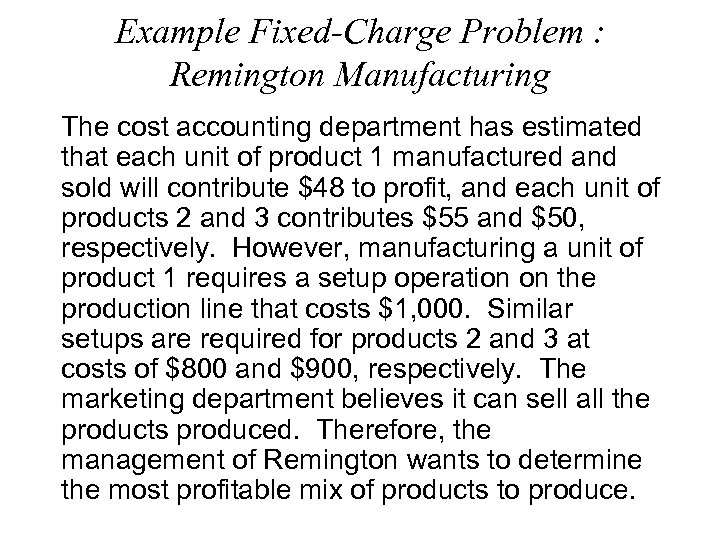Example Fixed-Charge Problem : Remington Manufacturing The cost accounting department has estimated that each unit of product 1 manufactured and sold will contribute \$48 to profit, and each unit of products 2 and 3 contributes \$55 and \$50, respectively. However, manufacturing a unit of product 1 requires a setup operation on the production line that costs \$1, 000. Similar setups are required for products 2 and 3 at costs of \$800 and \$900, respectively. The marketing department believes it can sell all the products produced. Therefore, the management of Remington wants to determine the most profitable mix of products to produce.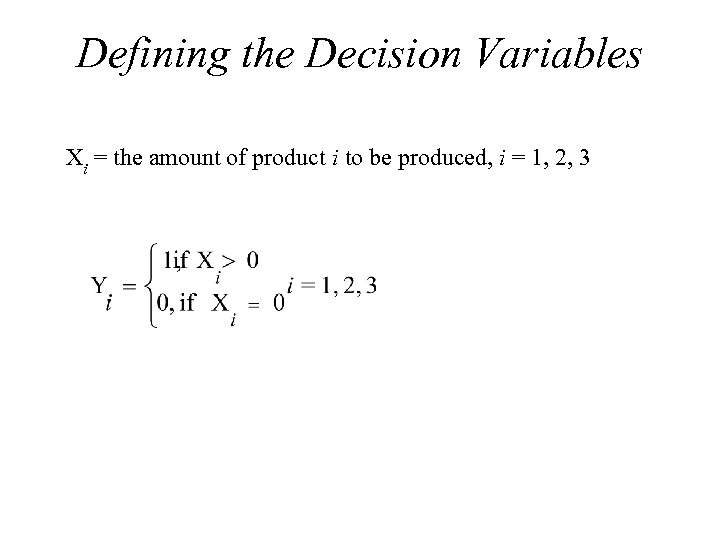Defining the Decision Variables Xi = the amount of product i to be produced, i = 1, 2, 3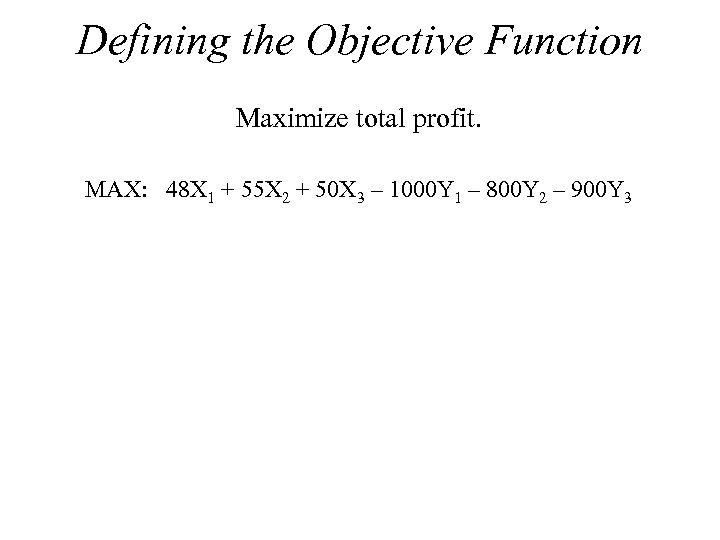Defining the Objective Function Maximize total profit. MAX: 48 X 1 + 55 X 2 + 50 X 3 – 1000 Y 1 – 800 Y 2 – 900 Y 3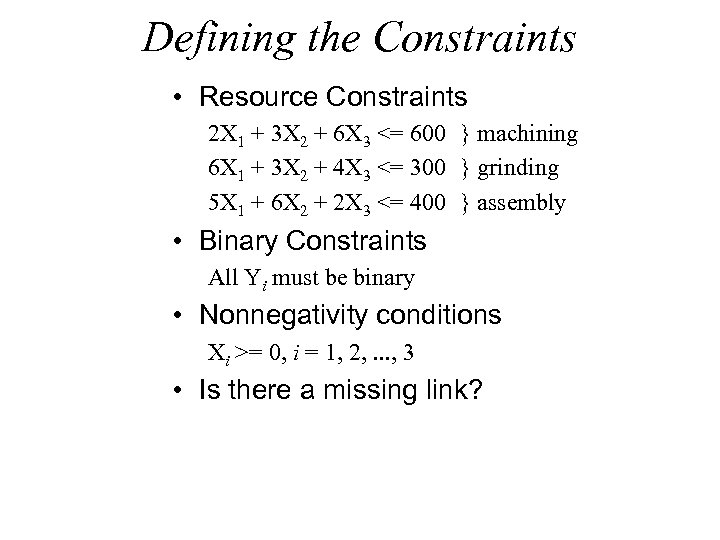Defining the Constraints • Resource Constraints 2 X 1 + 3 X 2 + 6 X 3 <= 600 } machining 6 X 1 + 3 X 2 + 4 X 3 <= 300 } grinding 5 X 1 + 6 X 2 + 2 X 3 <= 400 } assembly • Binary Constraints All Yi must be binary • Nonnegativity conditions Xi >= 0, i = 1, 2, . . . , 3 • Is there a missing link?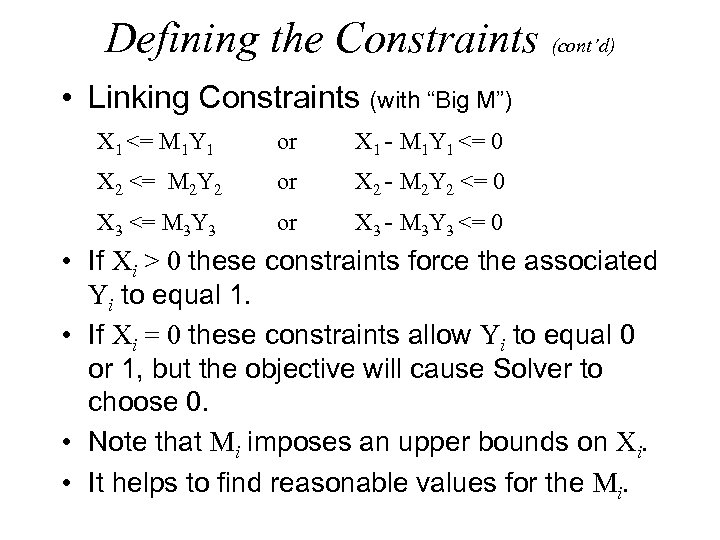Defining the Constraints (cont’d) • Linking Constraints (with “Big M”) X 1 <= M 1 Y 1 or X 1 - M 1 Y 1 <= 0 X 2 <= M 2 Y 2 or X 2 - M 2 Y 2 <= 0 X 3 <= M 3 Y 3 or X 3 - M 3 Y 3 <= 0 • If Xi > 0 these constraints force the associated Yi to equal 1. • If Xi = 0 these constraints allow Yi to equal 0 or 1, but the objective will cause Solver to choose 0. • Note that Mi imposes an upper bounds on Xi. • It helps to find reasonable values for the Mi.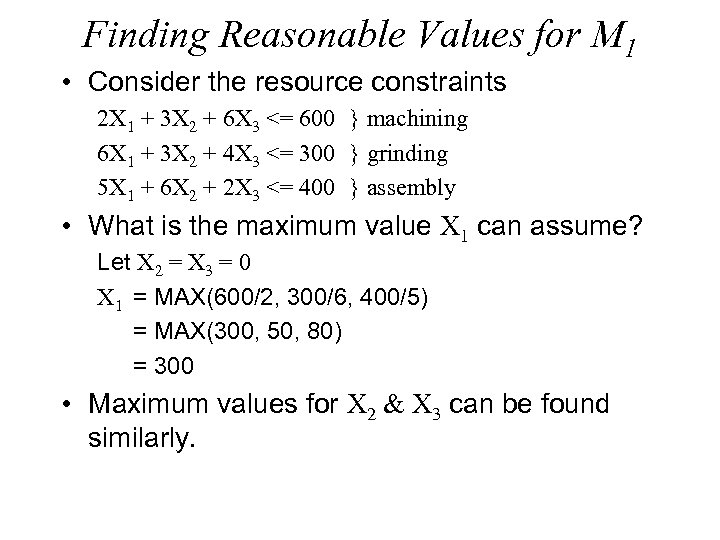Finding Reasonable Values for M 1 • Consider the resource constraints 2 X 1 + 3 X 2 + 6 X 3 <= 600 } machining 6 X 1 + 3 X 2 + 4 X 3 <= 300 } grinding 5 X 1 + 6 X 2 + 2 X 3 <= 400 } assembly • What is the maximum value X 1 can assume? Let X 2 = X 3 = 0 X 1 = MAX(600/2, 300/6, 400/5) = MAX(300, 50, 80) = 300 • Maximum values for X 2 & X 3 can be found similarly.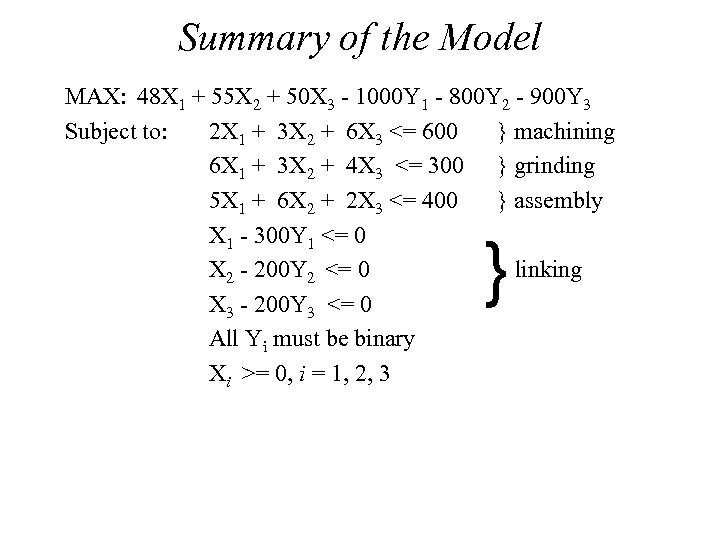Summary of the Model MAX: 48 X 1 + 55 X 2 + 50 X 3 - 1000 Y 1 - 800 Y 2 - 900 Y 3 Subject to: 2 X 1 + 3 X 2 + 6 X 3 <= 600 } machining 6 X 1 + 3 X 2 + 4 X 3 <= 300 } grinding 5 X 1 + 6 X 2 + 2 X 3 <= 400 } assembly X 1 - 300 Y 1 <= 0 X 2 - 200 Y 2 <= 0 linking X 3 - 200 Y 3 <= 0 All Yi must be binary Xi >= 0, i = 1, 2, 3 }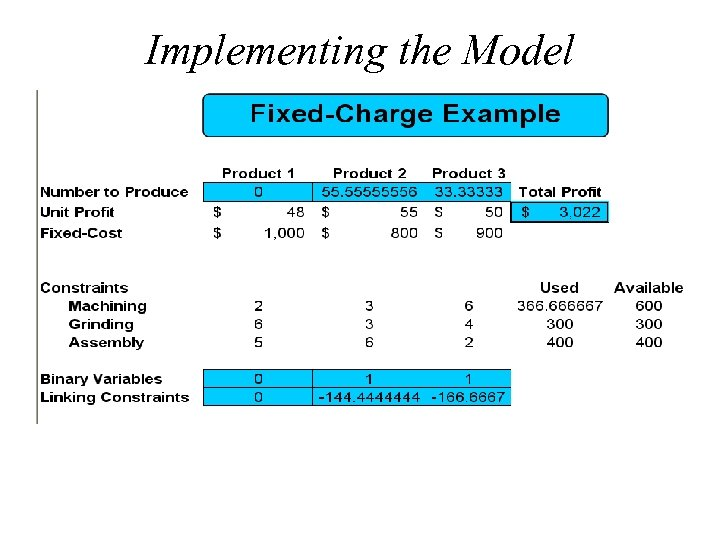Implementing the Model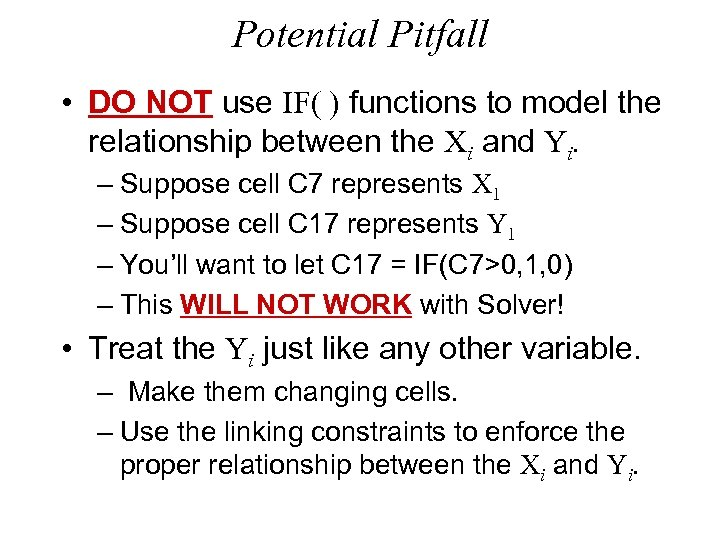Potential Pitfall • DO NOT use IF( ) functions to model the relationship between the Xi and Yi. – Suppose cell C 7 represents X 1 – Suppose cell C 17 represents Y 1 – You’ll want to let C 17 = IF(C 7>0, 1, 0) – This WILL NOT WORK with Solver! • Treat the Yi just like any other variable. – Make them changing cells. – Use the linking constraints to enforce the proper relationship between the Xi and Yi.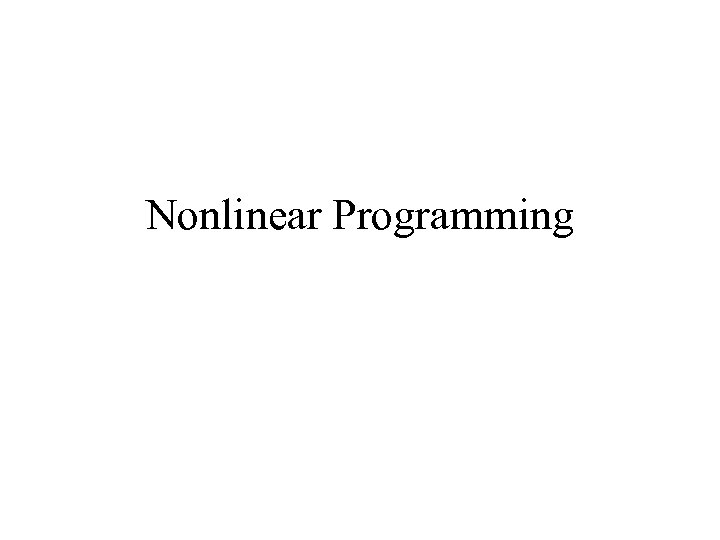Nonlinear Programming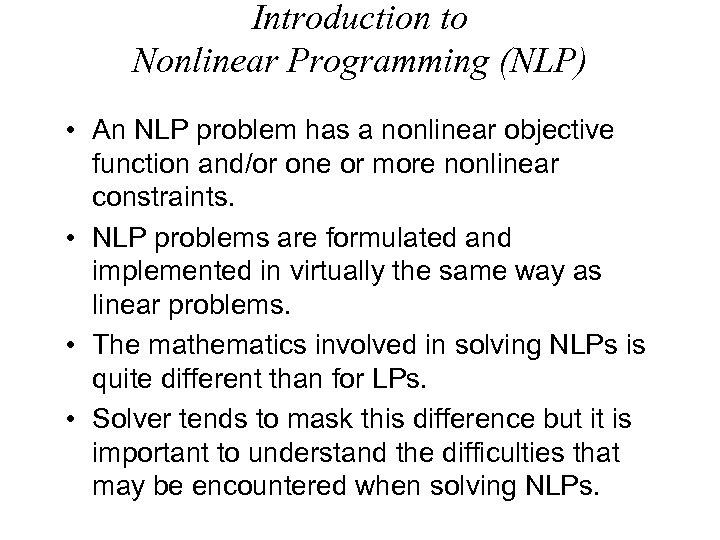Introduction to Nonlinear Programming (NLP) • An NLP problem has a nonlinear objective function and/or one or more nonlinear constraints. • NLP problems are formulated and implemented in virtually the same way as linear problems. • The mathematics involved in solving NLPs is quite different than for LPs. • Solver tends to mask this difference but it is important to understand the difficulties that may be encountered when solving NLPs.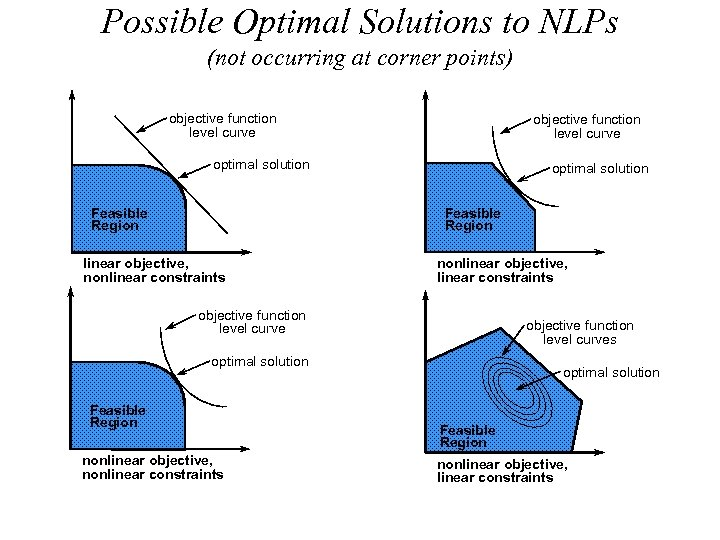Possible Optimal Solutions to NLPs (not occurring at corner points) objective function level curve optimal solution Feasible Region linear objective, nonlinear constraints nonlinear objective, linear constraints objective function level curves optimal solution Feasible Region nonlinear objective, nonlinear constraints optimal solution Feasible Region nonlinear objective, linear constraints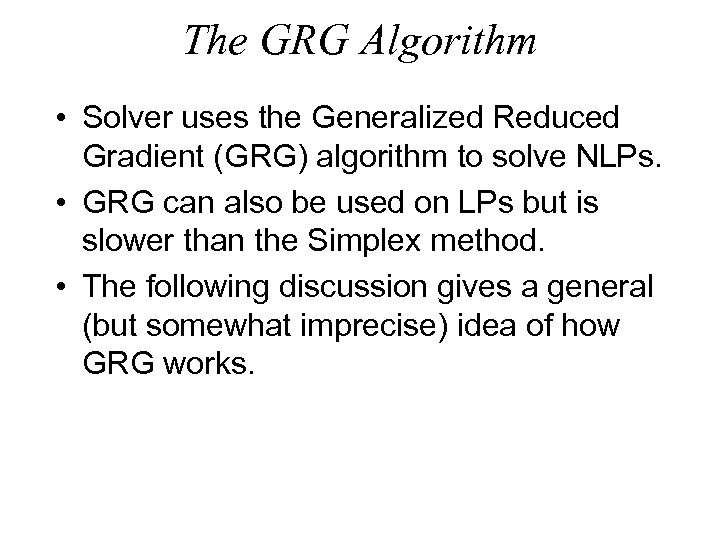The GRG Algorithm • Solver uses the Generalized Reduced Gradient (GRG) algorithm to solve NLPs. • GRG can also be used on LPs but is slower than the Simplex method. • The following discussion gives a general (but somewhat imprecise) idea of how GRG works.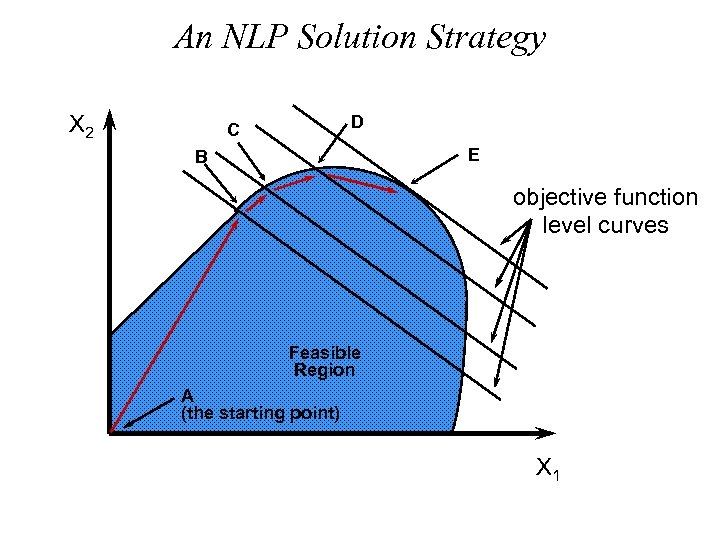An NLP Solution Strategy X 2 D C E B objective function level curves Feasible Region A (the starting point) X 1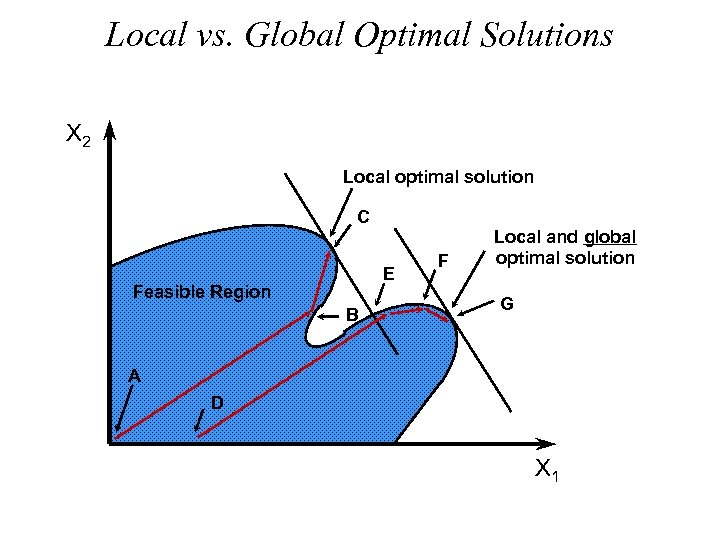Local vs. Global Optimal Solutions X 2 Local optimal solution C E Feasible Region B F Local and global optimal solution G A D X 1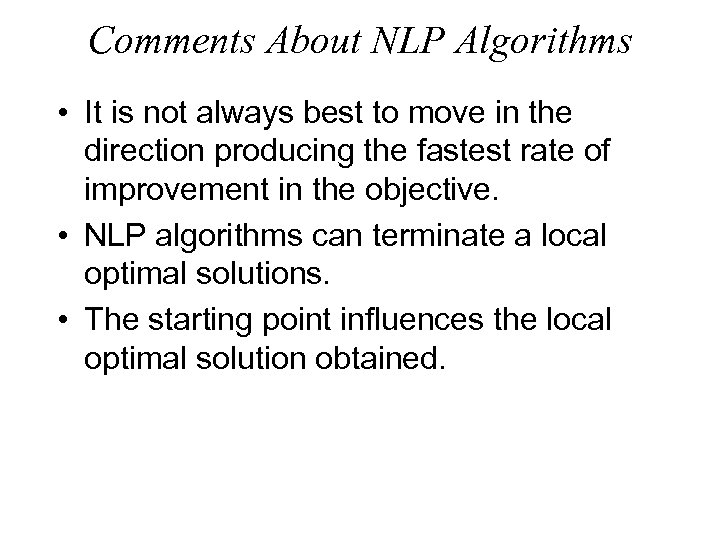Comments About NLP Algorithms • It is not always best to move in the direction producing the fastest rate of improvement in the objective. • NLP algorithms can terminate a local optimal solutions. • The starting point influences the local optimal solution obtained.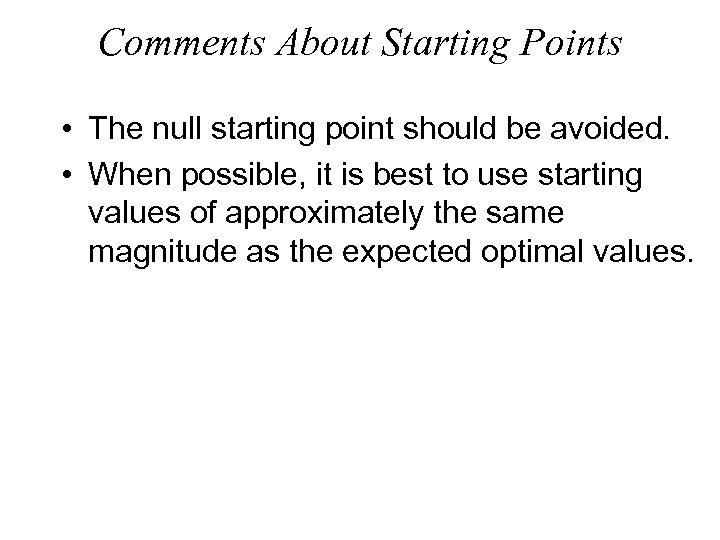Comments About Starting Points • The null starting point should be avoided. • When possible, it is best to use starting values of approximately the same magnitude as the expected optimal values.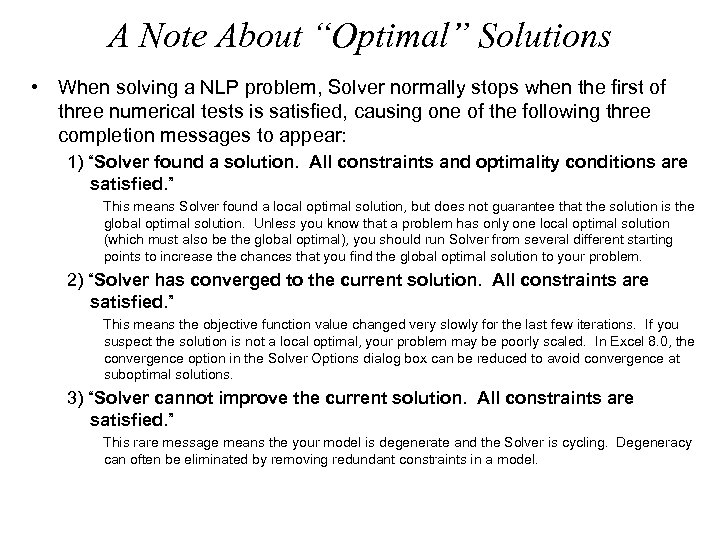A Note About “Optimal” Solutions • When solving a NLP problem, Solver normally stops when the first of three numerical tests is satisfied, causing one of the following three completion messages to appear: 1) “Solver found a solution. All constraints and optimality conditions are satisfied. ” This means Solver found a local optimal solution, but does not guarantee that the solution is the global optimal solution. Unless you know that a problem has only one local optimal solution (which must also be the global optimal), you should run Solver from several different starting points to increase the chances that you find the global optimal solution to your problem. 2) “Solver has converged to the current solution. All constraints are satisfied. ” This means the objective function value changed very slowly for the last few iterations. If you suspect the solution is not a local optimal, your problem may be poorly scaled. In Excel 8. 0, the convergence option in the Solver Options dialog box can be reduced to avoid convergence at suboptimal solutions. 3) “Solver cannot improve the current solution. All constraints are satisfied. ” This rare message means the your model is degenerate and the Solver is cycling. Degeneracy can often be eliminated by removing redundant constraints in a model.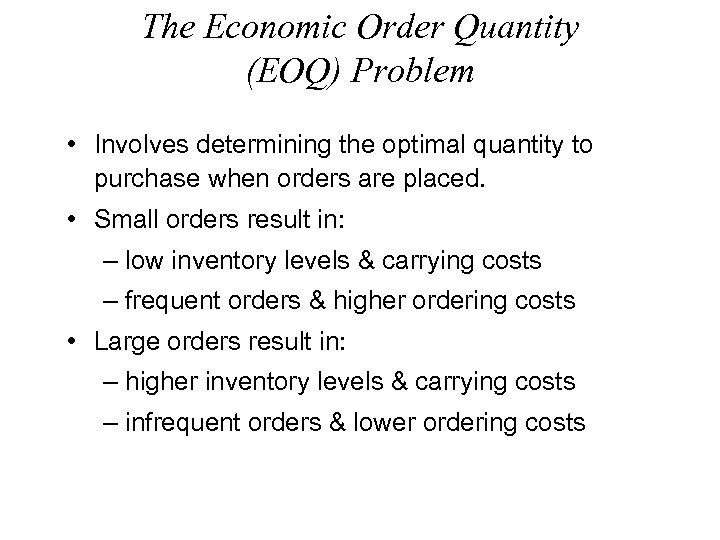The Economic Order Quantity (EOQ) Problem • Involves determining the optimal quantity to purchase when orders are placed. • Small orders result in: – low inventory levels & carrying costs – frequent orders & higher ordering costs • Large orders result in: – higher inventory levels & carrying costs – infrequent orders & lower ordering costs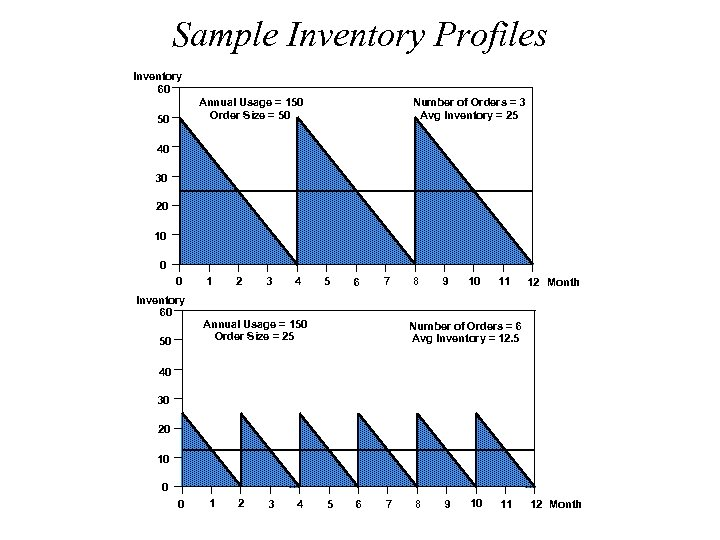Sample Inventory Profiles Inventory 60 Annual Usage = 150 Order Size = 50 50 Number of Orders = 3 Avg Inventory = 25 40 30 20 10 0 0 Inventory 60 50 1 2 3 4 5 6 7 Annual Usage = 150 Order Size = 25 8 9 10 11 12 Month Number of Orders = 6 Avg Inventory = 12. 5 40 30 20 10 0 0 1 2 3 4 5 6 7 8 9 10 11 12 Month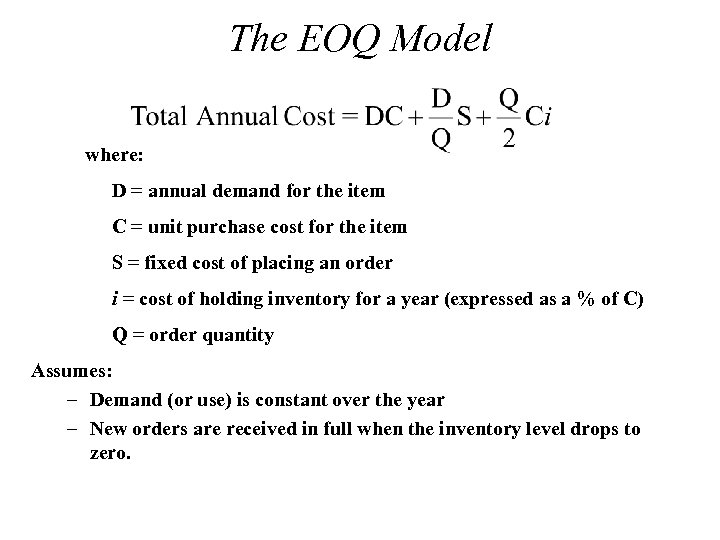The EOQ Model where: D = annual demand for the item C = unit purchase cost for the item S = fixed cost of placing an order i = cost of holding inventory for a year (expressed as a % of C) Q = order quantity Assumes: – Demand (or use) is constant over the year – New orders are received in full when the inventory level drops to zero.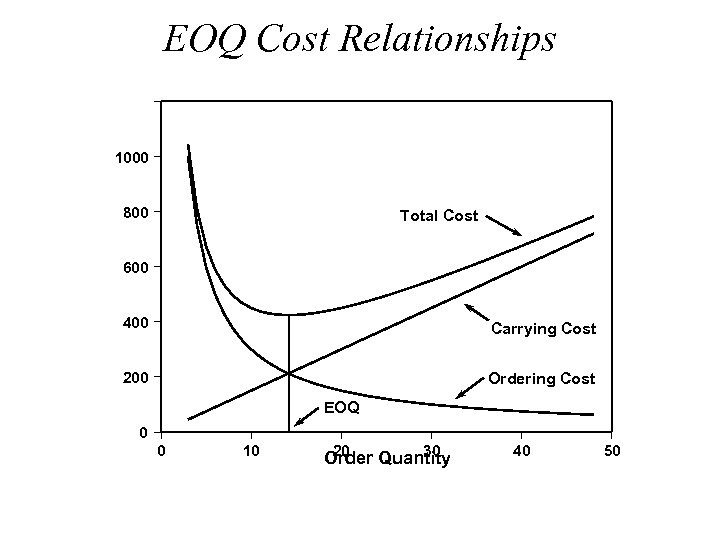EOQ Cost Relationships \$ 1000 800 Total Cost 600 400 Carrying Cost 200 Ordering Cost EOQ 0 0 10 20 30 Order Quantity 40 50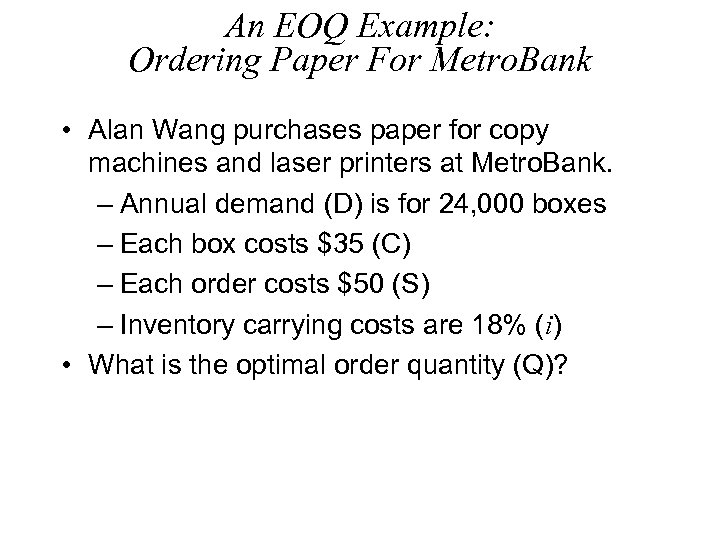An EOQ Example: Ordering Paper For Metro. Bank • Alan Wang purchases paper for copy machines and laser printers at Metro. Bank. – Annual demand (D) is for 24, 000 boxes – Each box costs \$35 (C) – Each order costs \$50 (S) – Inventory carrying costs are 18% (i) • What is the optimal order quantity (Q)?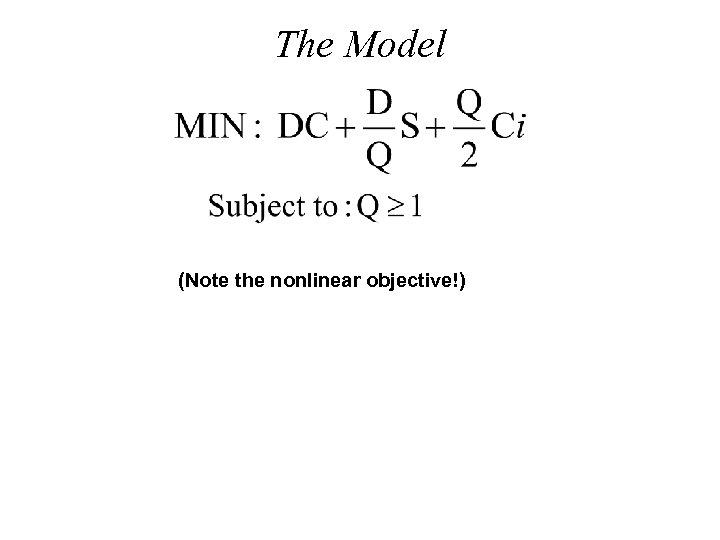The Model (Note the nonlinear objective!)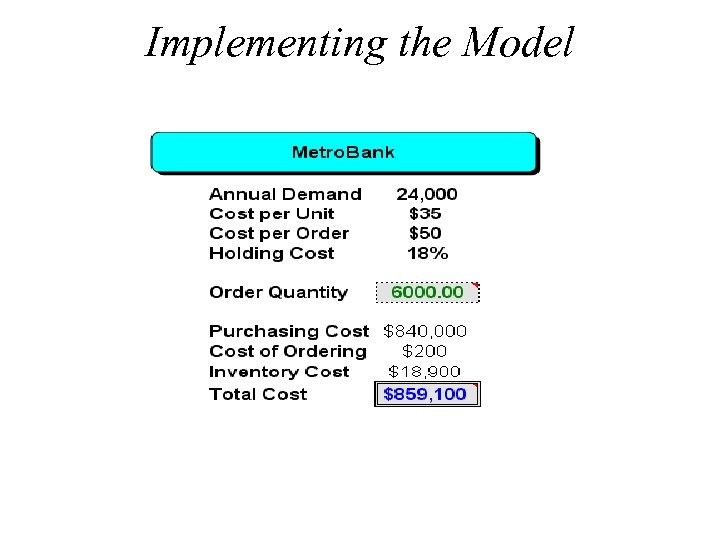Implementing the Model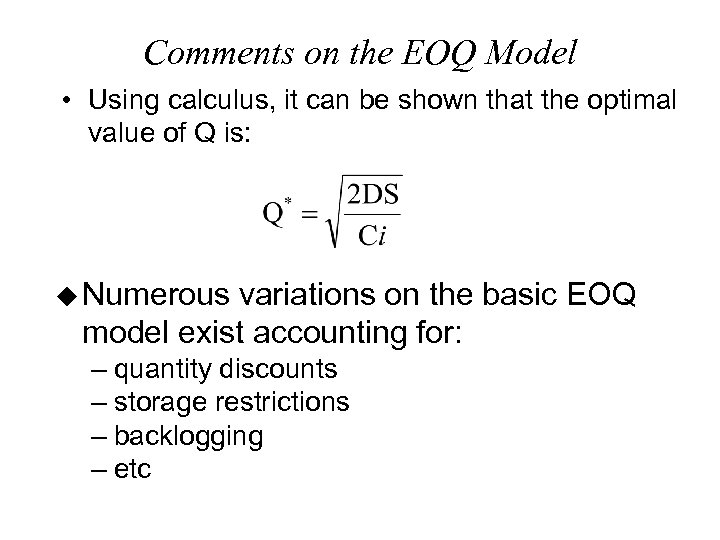Comments on the EOQ Model • Using calculus, it can be shown that the optimal value of Q is: u Numerous variations on the basic EOQ model exist accounting for: – quantity discounts – storage restrictions – backlogging – etc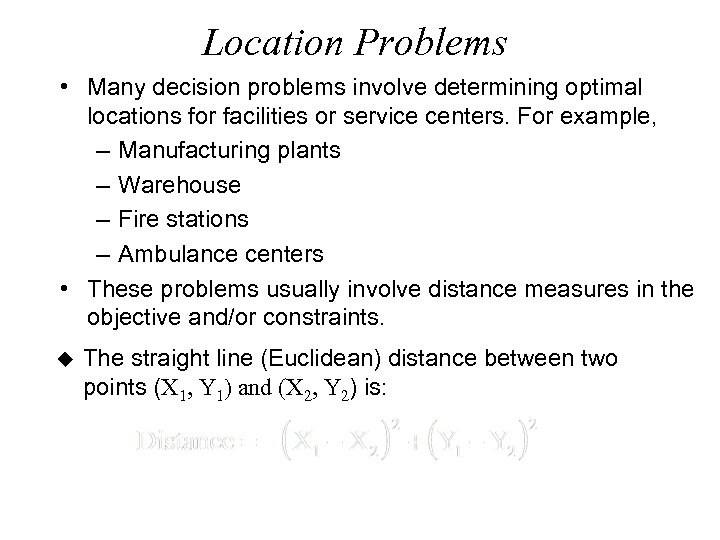Location Problems • Many decision problems involve determining optimal locations for facilities or service centers. For example, – Manufacturing plants – Warehouse – Fire stations – Ambulance centers • These problems usually involve distance measures in the objective and/or constraints. u The straight line (Euclidean) distance between two points (X 1, Y 1) and (X 2, Y 2) is: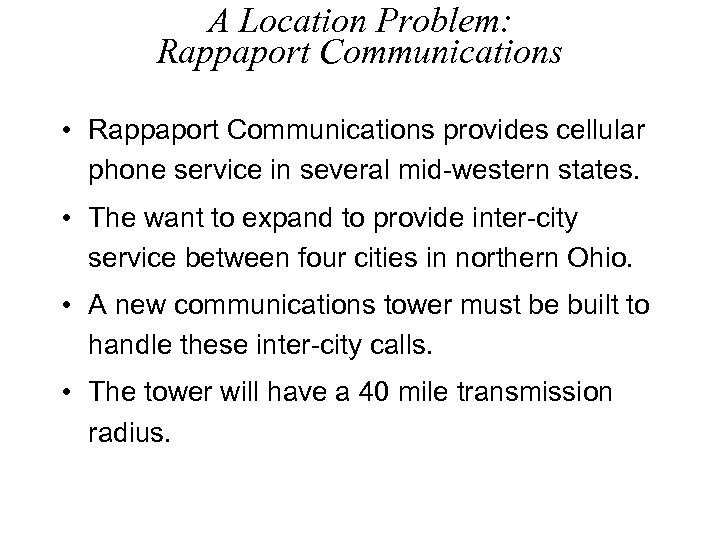A Location Problem: Rappaport Communications • Rappaport Communications provides cellular phone service in several mid-western states. • The want to expand to provide inter-city service between four cities in northern Ohio. • A new communications tower must be built to handle these inter-city calls. • The tower will have a 40 mile transmission radius.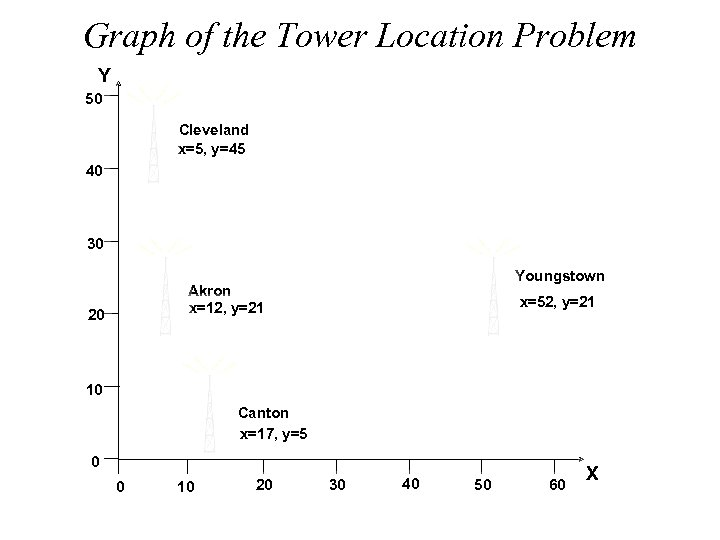Graph of the Tower Location Problem Y 50 Cleveland x=5, y=45 40 30 Youngstown Akron x=12, y=21 20 x=52, y=21 10 Canton x=17, y=5 0 0 10 20 30 40 50 60 X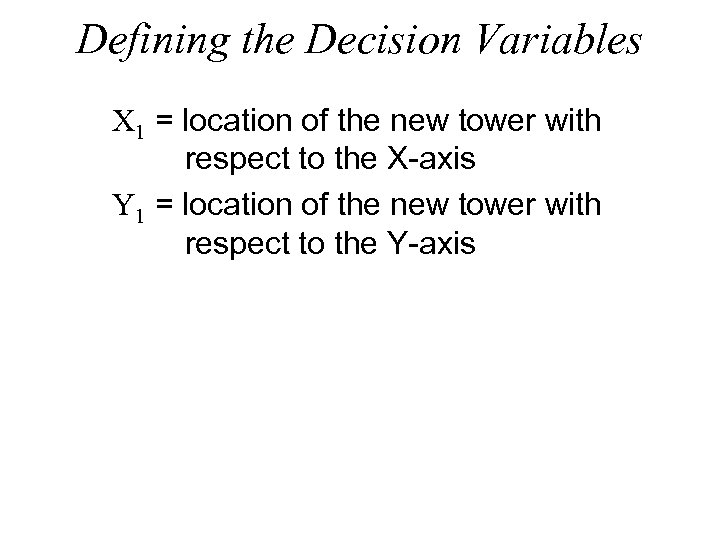Defining the Decision Variables X 1 = location of the new tower with respect to the X-axis Y 1 = location of the new tower with respect to the Y-axis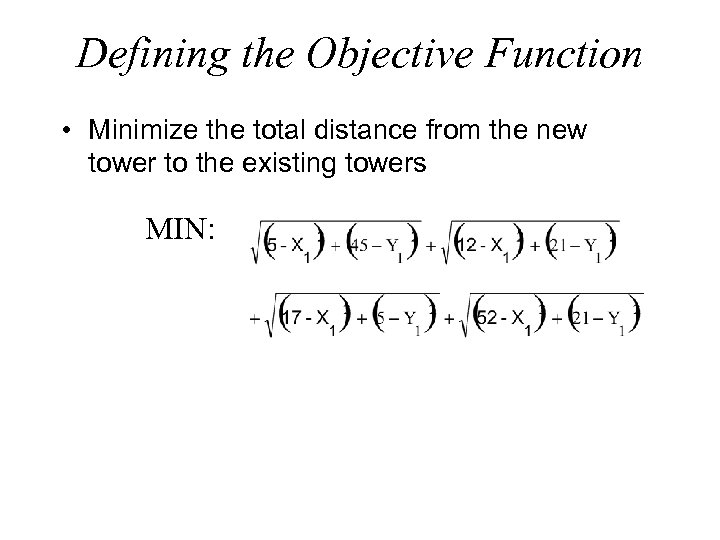Defining the Objective Function • Minimize the total distance from the new tower to the existing towers MIN: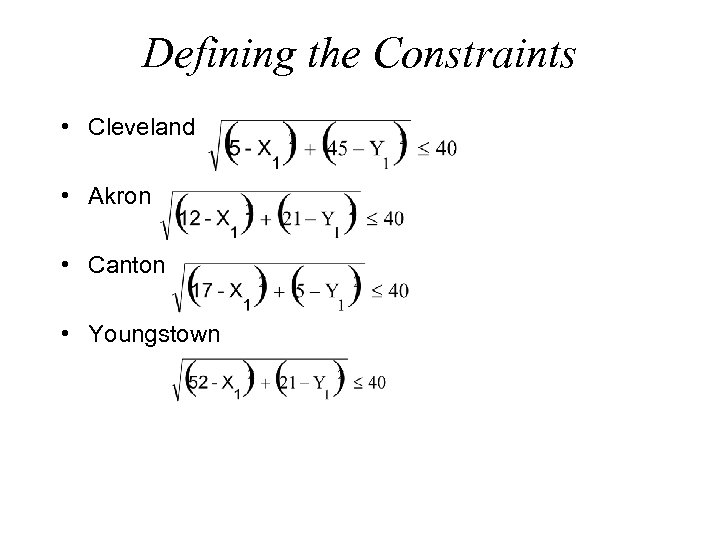Defining the Constraints • Cleveland • Akron • Canton • Youngstown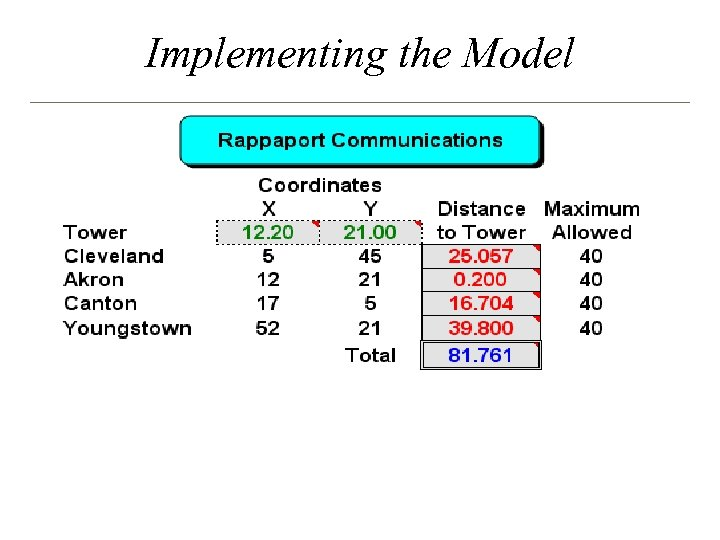Implementing the Model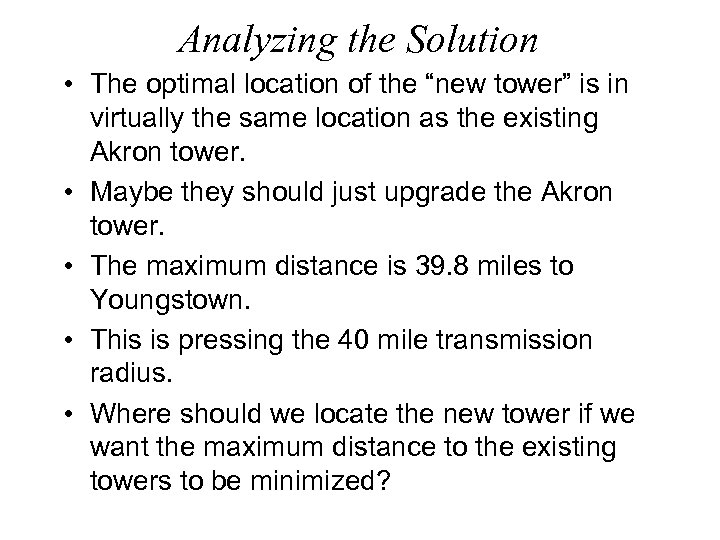Analyzing the Solution • The optimal location of the “new tower” is in virtually the same location as the existing Akron tower. • Maybe they should just upgrade the Akron tower. • The maximum distance is 39. 8 miles to Youngstown. • This is pressing the 40 mile transmission radius. • Where should we locate the new tower if we want the maximum distance to the existing towers to be minimized?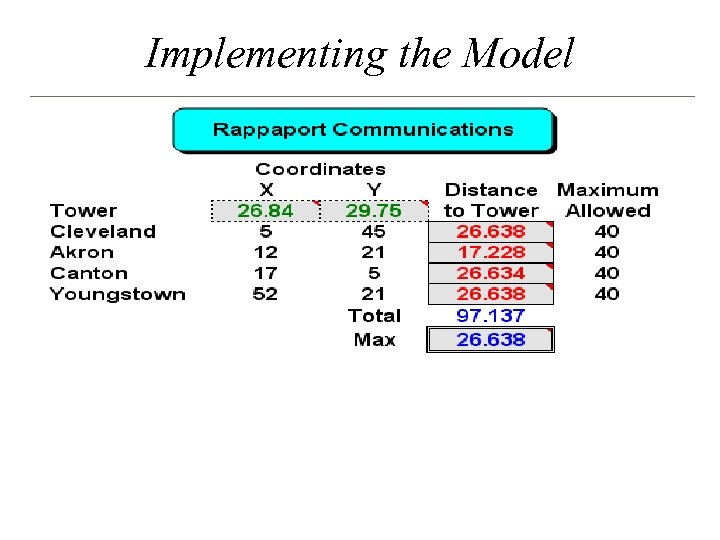Implementing the Model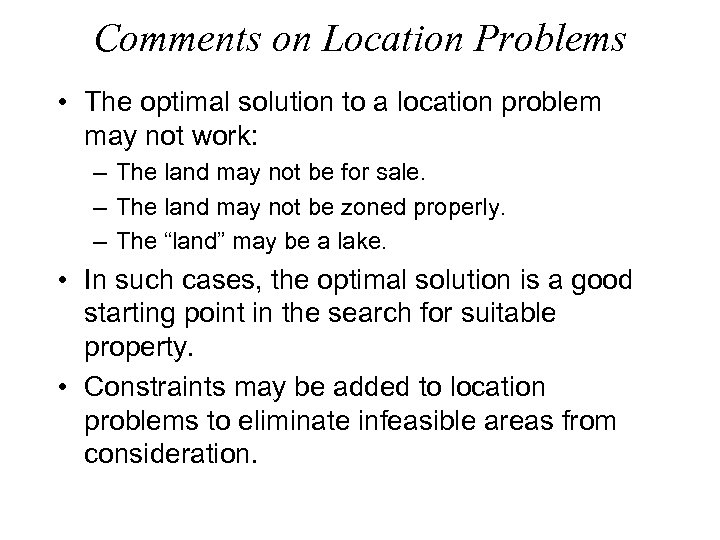Comments on Location Problems • The optimal solution to a location problem may not work: – The land may not be for sale. – The land may not be zoned properly. – The “land” may be a lake. • In such cases, the optimal solution is a good starting point in the search for suitable property. • Constraints may be added to location problems to eliminate infeasible areas from consideration.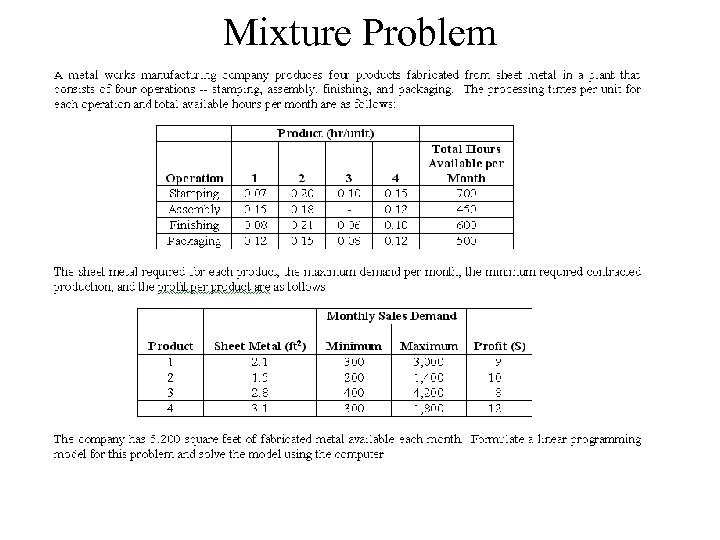Mixture ProblemFormulate a model for this problem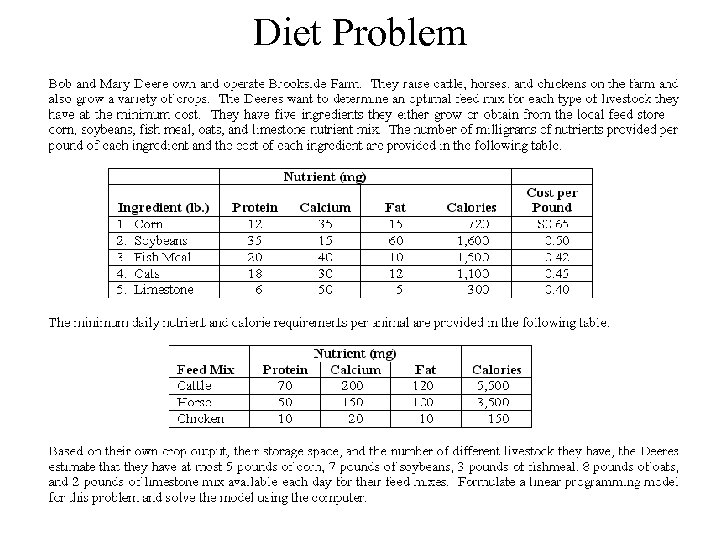Diet ProblemFormulate a model for this problem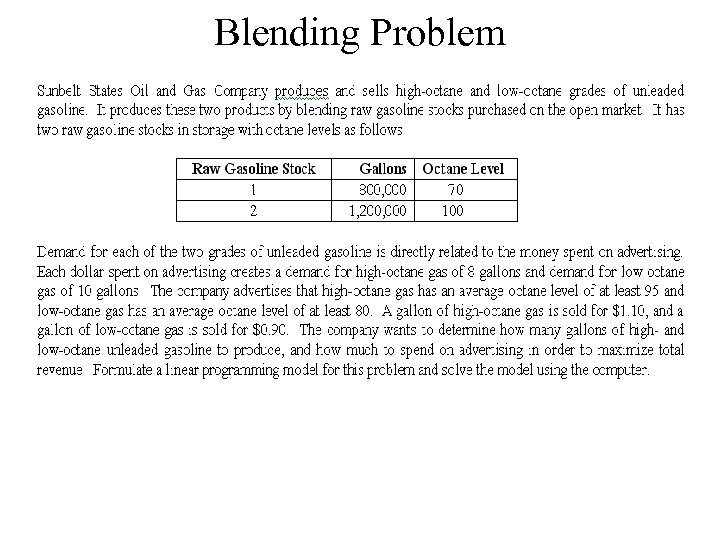Blending ProblemFormulate a model for this problem